### Home > CALC > Chapter 2 > Lesson 2.3.3 > Problem2-126

2-126.
1. The rate of customers who pass through the checkout stand at a grocery store depends on the time of day. Assume the rate follows the following piecewise function, where x represents the time of day (in hours) after 9:00 a.m. and f(x) is the number of customers served per hour. Homework Help ✎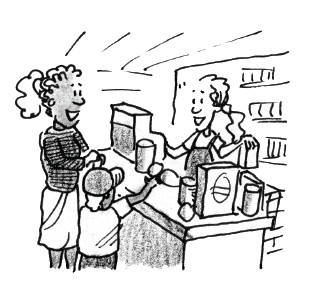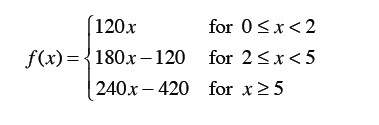1. Graph the function and name its domain and range.

2. Is this function continuous?

3. Find A(f, 0 ≤ x ≤ 8). What are the units of this area? What does this area represent?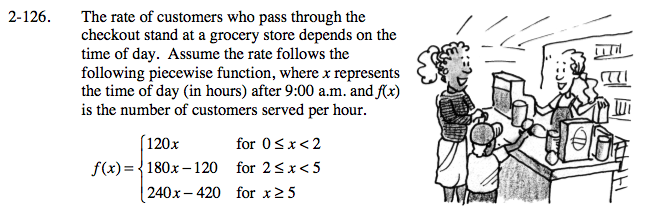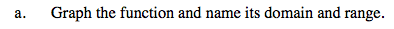Your sketch should have three linear pieces.

The domain is given by the piecewise function above.

The range can be seen on the graph. What is the highest and lowest y-value? Are there any holes or jumps on that interval?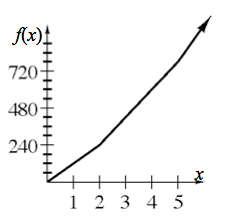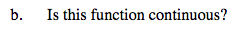We know that the graph has a starting point (0,0) so, it could only be continuous when x > 0.

Be mindful of the 3 conditions of continuity: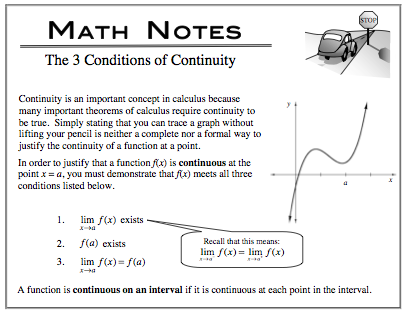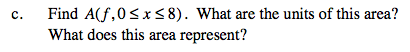Area = k(base)(height), where k is some constant. The base is determined by the units on the x-axis. The height is determined by the units on the y-axis. Multiply.